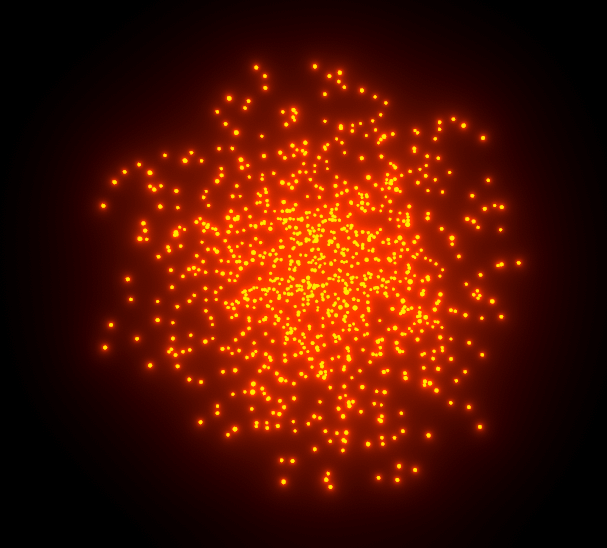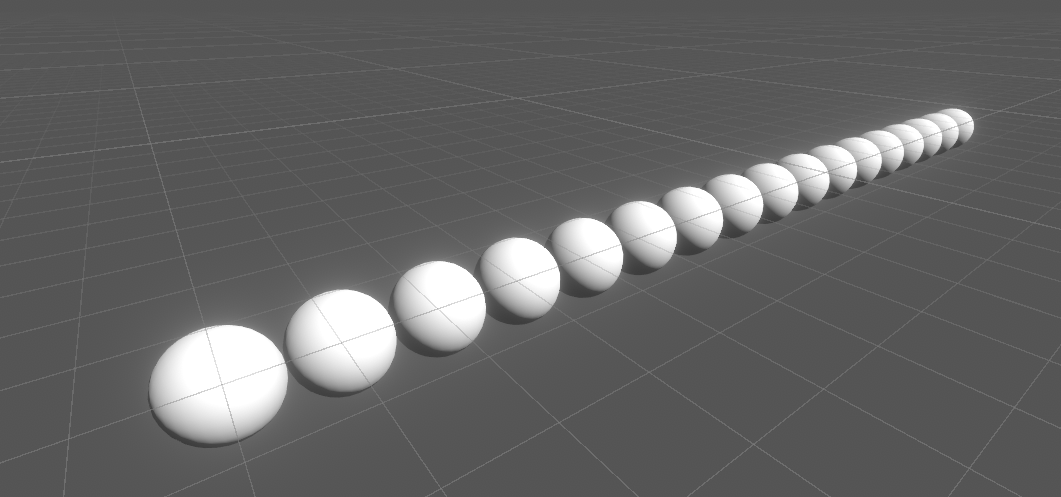So far we always used shaders to render with a fixed pipeline into textures, but modern graphics card can do way more than just that (sometimes they’re also referred to as GPGPU for “general purpose graphics processing unit” because of that). To do things that arent in the fix pipeline we’re using so far we have to use compute shaders.

If you’re asking yourself why we’d do that, the CPU is performant enough, especially once we use multithreading then I’m here to tell you that you’re 100% correct. You don’t need the GPU for you non graphics tasks. Using it will give you way more confusing error behaviour. The debugger wont give you as nice information and you can’t have breakpoints. Optimizing is weirder since you always have to think about what data you’re pushing around. The paralell nature of the GPU forces you to think way differently… If you don’t need compute shaders, especially when you’re not experienced, think about why you want to and if it’s making your more work than its worth.

If you’re still here, lets get going. In Unity you can check whether your GPU supports compute shaders by checking SystemInfo.supportsComputeShaders.We can get a simple compute shader by just doing `rclick>Create>Shader>Compute Shader`. The default shader does a few calculations to write a pattern into a texture, but for this tutorial I want to go one step simpler and just write positions into an array.

In compute land an array we can write into is a `RWStructuredBuffer` with a certain type (`StructuredBuffer` is a array we can only read from). This type can be next to any type including vectors or structs. In our case we’ll use a float3 vector.

The function that calculates stuff is called the kernel. We have to add a `numthreads` attribute in front of the kernel and the kernel takes in one argument which tells us which iteration of the kernel we’re in. In our case we define the number of threads as 64 threads in the `x` dimension and 1 in both `y` and `z`. Those values should work for most platforms that support compute shaders and its in 1 dimension so we dont have to do any rethinking when writing to our 1d array. The input argument is also 3d for that reason but for now we only care about the x part. We do have to mark it as `SV_DispatchThreadID` though so the correct value is assigned to it.

To tell unity which functions are just regular functions that are called from somewhere else and which ones are the kernel functions we add a pragma statement, so `#pragma kernel <functionname>`. Its possible to have multiple kernels per shader file.

``````// Each #kernel tells which function to compile; you can have many kernels
#pragma kernel Spheres

//variables
RWStructuredBuffer<float3> Result;

void Spheres (uint3 id : SV_DispatchThreadID)
{
}
``````

As a starting point I’ll just let the program write a id,0,0 positions in the buffer we set up.

``````[numthreads(64,1,1)]
void Spheres (uint3 id : SV_DispatchThreadID)
{
Result[id.x] = float3(id.x, 0, 0);
}
``````

Unlike graphics shaders compute shaders can’t just be assigned to a material and via that to a object. Instead we trigger the execution ourselves from c# code.

In the C# component we create we reference our compute shader via a variable of the type `ComputeShader`. We also create a integer to store the identifier of our kernel after getting it once in the start method. To get the kernel identifier we call the `FindKernel(<kernelname>)` function on our compute shader. And after getting the kernel identifier we can use it to get the size of each thread group (thats equal to our `numthreads` in the shader). We store the x size in a variable and discard the others by passing in `_` as a output variable.

Lets also make the length of the buffer we’re filling a public property so we can change that in the future. With that information available we can also create the gpu array we’ll write to as a `ComputeBuffer`. Its constructor takes in the amount of elements as the first parameter and the size of its content as the second parameter. Since we’re using float3 on the shader side, we can get the size(in bytes) of a float with the `sizeof` function and multiply the result by 3(getting the size of a Vector3 or a float3 of the new mathematics lib is also possible, but only in a unsafe context and that sounds scary(it isn’t really, but whatever)). Paralell to the ComputeBuffer lets also create a regular Vector3 array of the same size, we’ll use it later to copy the data back to the ram where we can use it. If we want to write clean code (we kinda do) we should also call `Dispose` on our buffer when the component is destroyed so unity can do garbage collection, so lets add that to the OnDestroy method.

With all of that set up we can use the shader in the update method. First we declare set the buffer of the shader to be our buffer, this buffer is set per kernel so we also have to pass in our kernel identifier. To dispatch the shader we first calculate how many threadgroups we need, in our case we want the amount of threads to be the length of the array, so the thread groups should be that amount divided by the thread size rounded up. When dealing with integers the easiest way of doing a division and getting the rounded up result is to add the divisor minus one before the division, that adds 1 to the result unless the dividend is a exact multiple of the divisor. After thats done we can dispatch the shader and tell it how many thread groups it should run (the amount we just calculated in `x`, and 1 in `y` and `z`). And then we can already get the data out of the buffer into ram we can work with in C# with the aptly named `GetData` function.

``````public class BasicComputeSpheres : MonoBehaviour
{
public int SphereAmount = 17;

ComputeBuffer resultBuffer;
int kernel;
Vector3[] output;

void Start()
{
//program we're executing

//buffer on the gpu in the ram
resultBuffer = new ComputeBuffer(SphereAmount, sizeof(float) * 3);
output = new Vector3[SphereAmount];
}

void Update()
{
resultBuffer.GetData(output);
}

void OnDestroy()
{
resultBuffer.Dispose();
}
}
``````

Now we have the data but we can’t see it. There are ways of rendering the buffer directly on the GPU, but this is not the tutorial for that, instead I decided to instantiate a bunch of prefabs and use them for visualisation. The instanced transforms are saved in a private array which is created and filled with new prefab instances in the start method. The length of the array is the same length of the buffer.

In update method we then copy the positions from the output struct to the local position of the objects.

``````// in start method

//spheres we use for visualisation
instances = new Transform[SphereAmount];
for (int i = 0; i < SphereAmount; i++)
{
instances[i] = Instantiate(Prefab, transform).transform;
}
``````
``````//in update method
for (int i = 0; i < instances.Length; i++)
instances[i].localPosition = output[i];
``````## A tiny bit more complex Compute Shader

The rest of this is just to make stuff look nice, its just plain hlsl like in all my other tutorials.

In the Compute Shader I first include the functions from my tutorial on randomness and add a new variable called time. In the kernel function I get a random vector(based on the kernel index), normalize it and get it to a random length between 0.1 and 1 (if I let it go too short bad math can happen too easily and some points become NaN). Then I generate a new vector thats orthogonal to that one by taking the cross product between the vector and a different random vector(this isn’t guaranteed to work, if the vectors are paralell the cross product is `(0,0,0)` and can’t e normalized, but it works well enough) and give its length the same treatment to make it be between 0.1 and 0.9. The random looking numbers I add to the inputs are to avoid some of the symmetry so not all random functions return the same result. Then I get a time variable by multiplying the time by 2(that 2 could be a uniform value if you want to adjust the speed manually) and give it a offset by a random value multiplied by some big-ish odd number.

Those values can then be combined by multiplying one of the vectors by the sine of the time and the other by the cosine of the time and adding the 2 results. I then also multiplied it by 20 to make it bigger, but you should also consider using a property settable from outside here(do as I say not as I do).

``````// Each #kernel tells which function to compile; you can have many kernels
#pragma kernel Spheres

#include "Random.cginc"

//variables
RWStructuredBuffer<float3> Result;
uniform float Time;

void Spheres (uint3 id : SV_DispatchThreadID)
{
//generate 2 orthogonal vectors
float3 baseDir = normalize(rand1dTo3d(id.x) - 0.5) * (rand1dTo1d(id.x)*0.9+0.1);
float3 orthogonal = normalize(cross(baseDir, rand1dTo3d(id.x + 7.1393) - 0.5)) * (rand1dTo1d(id.x+3.7443)*0.9+0.1);
//scale the time and give it a random offset
float scaledTime = Time * 2 + rand1dTo1d(id.x) * 712.131234;
//calculate a vector based on vectors
float3 dir = baseDir * sin(scaledTime) + orthogonal * cos(scaledTime);
Result[id.x] = dir * 20;
}
``````

Then all thats missing is passing in the time from the C# code and you should have a nice orbiting swarm.

``````Shader.SetFloat("Time", Time.time);
``````

With a emissive material and a simple bloom from the postprocessing stack it can look like this:## Source

``````// Each #kernel tells which function to compile; you can have many kernels
#pragma kernel Spheres

#include "Random.cginc"

//variables
RWStructuredBuffer<float3> Result;
uniform float Time;

void Spheres (uint3 id : SV_DispatchThreadID)
{
//generate 2 orthogonal vectors
float3 baseDir = normalize(rand1dTo3d(id.x) - 0.5) * (rand1dTo1d(id.x)*0.9+0.1);
float3 orthogonal = normalize(cross(baseDir, rand1dTo3d(id.x + 7.1393) - 0.5)) * (rand1dTo1d(id.x+3.7443)*0.9+0.1);
//scale the time and give it a random offset
float scaledTime = Time * 2 + rand1dTo1d(id.x) * 712.131234;
//calculate a vector based on vectors
float3 dir = baseDir * sin(scaledTime) + orthogonal * cos(scaledTime);
Result[id.x] = dir * 20;
}
``````
``````using System;
using System.Collections;
using System.Collections.Generic;
using UnityEngine;

public class BasicComputeSpheres : MonoBehaviour
{
public int SphereAmount = 17;

public GameObject Prefab;

ComputeBuffer resultBuffer;
int kernel;
Vector3[] output;

Transform[] instances;

void Start()
{
//program we're executing

//buffer on the gpu in the ram
resultBuffer = new ComputeBuffer(SphereAmount, sizeof(float) * 3);
output = new Vector3[SphereAmount];

//spheres we use for visualisation
instances = new Transform[SphereAmount];
for (int i = 0; i < SphereAmount; i++)
{
instances[i] = Instantiate(Prefab, transform).transform;
}
}

void Update()
{
resultBuffer.GetData(output);

for (int i = 0; i < instances.Length; i++)
instances[i].localPosition = output[i];
}

void OnDestroy()
{
resultBuffer.Dispose();
}
}
``````

You can also find me on twitter at @totallyRonja. If you liked my tutorial and want to support me you can do that on Patreon (patreon.com/RonjaTutorials) or Ko-Fi (ko-fi.com/RonjaTutorials).